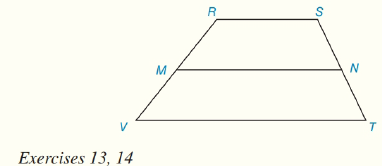Chapter 4.CT, Problem 13CTElementary Geometry For College St...

7th Edition
Alexander + 2 others
ISBN: 9781337614085

Solutions

Chapter
SectionElementary Geometry For College St...

7th Edition
Alexander + 2 others
ISBN: 9781337614085
Textbook Problem

In trapezoid RSTV, R S ¯ ∥ V T ¯ and M N ¯ is the median, Find the length MN if RS=12.4 in. and VT=16.2 in.To determine

To find:

The length of MN.

Explanation

Given:

Trapezoid RSTV,

RS¯VT¯ and MN¯

Calculation:

From the figure,

RS¯ and VT¯ are the bases of the trapezoid

Still sussing out bartleby?

Check out a sample textbook solution.

See a sample solution

The Solution to Your Study Problems

Bartleby provides explanations to thousands of textbook problems written by our experts, many with advanced degrees!

Get Started

Find more solutions based on key concepts